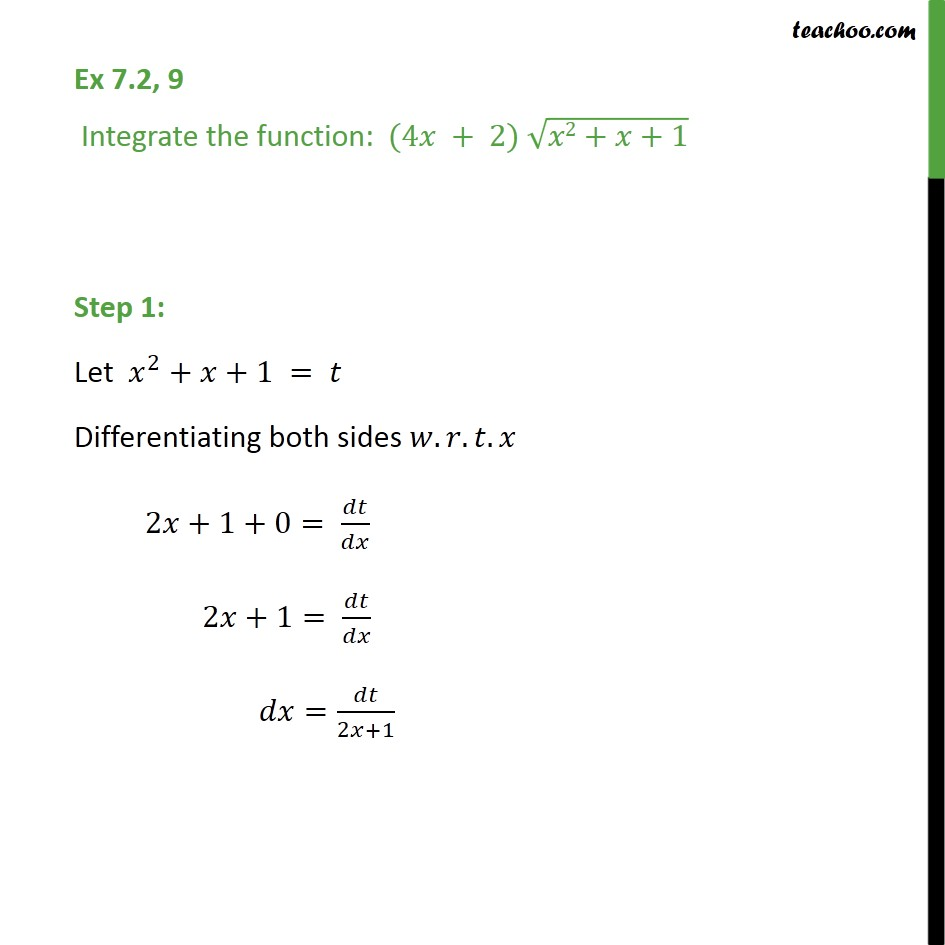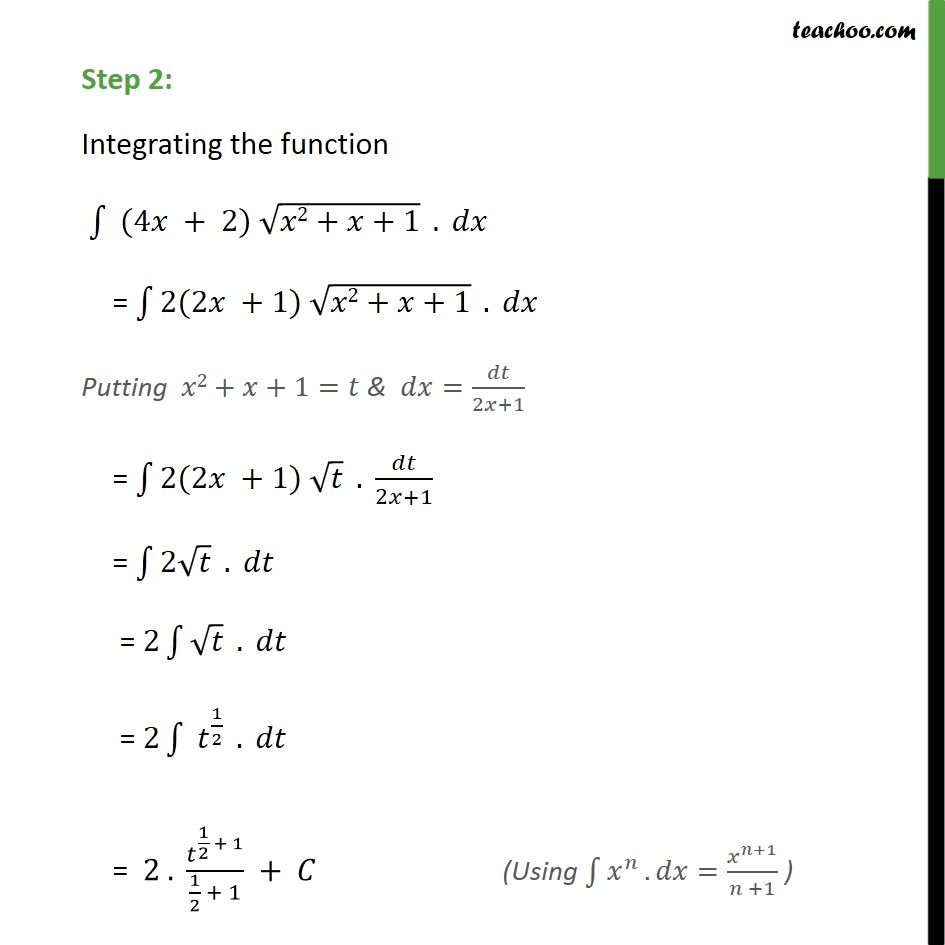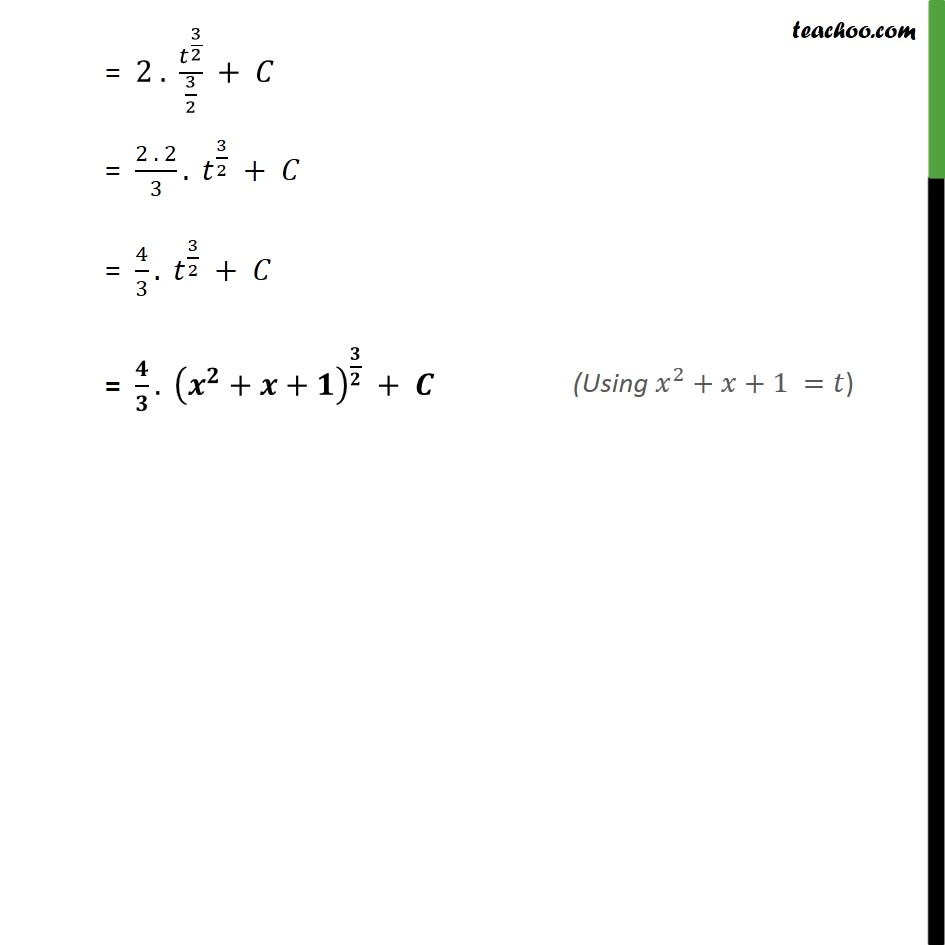Ex 7.2

Chapter 7 Class 12 Integrals
Serial order wiseSolve all your doubts with Teachoo Black (new monthly pack available now!)

### Transcript

Ex 7.2, 9 Integrate the function: (4 + 2) 2+ +1 Step 1: Let 2 + +1 = Differentiating both sides . . . 2 +1+0= 2 +1= = 2 +1 Step 2: Integrating the function (4 + 2) 2+ +1 . = 2(2 +1) 2+ +1 . Putting 2+ +1= & = 2 +1 = 2(2 +1) . 2 +1 = 2 . = 2 . = 2 1 2 . = 2 . 1 2 + 1 1 2 + 1 + = 2 . 3 2 3 2 + = 2 . 2 3 . 3 2 + = 4 3 . 3 2 + = . + + +